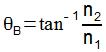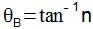Optics

# Brewster’s Law

Brewster’s law demonstrates the formation of the so-called Brewster angle which, added to the refraction angle, must result in a right angle.

Reflection of light occurs when light rays that fall on a given surface, forming a certain angle θ with the normal line , are returned to the source medium. In reflection, the velocity, frequency and wavelength , characteristic of incident light, are not changed.

The refraction of light , in turn, happens when the propagation medium is changed, causing the light rays to undergo a change in speed. In refraction, the frequency of the light wave is maintained, but the speed is changed as a result of the change in wavelength .

Brewster’s Law

When the sum of the angle of incidence (or reflection) and the angle of refraction, for a given ray of light, results in a right angle (90°), the angle of incidence is called the Brewster angle ( θ B ). In the image below, θ 1 is the angle of incidence and θ 2 is the angle of refraction of a ray of light, which strikes the separating surface between the media with refractive indices n 1 and n 2 .

If the sum θ 1 + θ 2 results in 90°, we say that the angle of incidence θ 1 is Brewster’s angle.

The equation below shows the definition of the Brewster angle as a function of the refractive indices of the media involved.If the incident rays propagate in air or in a vacuum, it can be said that n 1 = 1, therefore, the general form of the above equation, known as Brewster’s law, will be:In this equation, n represents the medium with refractive index n 2 , the propagation medium to which light travels.

By focusing exactly on the Brewster angle, light can undergo reflection polarization , in which the reflected light rays will have only one of the components of the electric field. When looking at sunlight reflecting off surfaces such as glass and water, you can usually see a brighter spot. In this region, the light is being polarized by reflection, completely perpendicular to the plane of incidence.